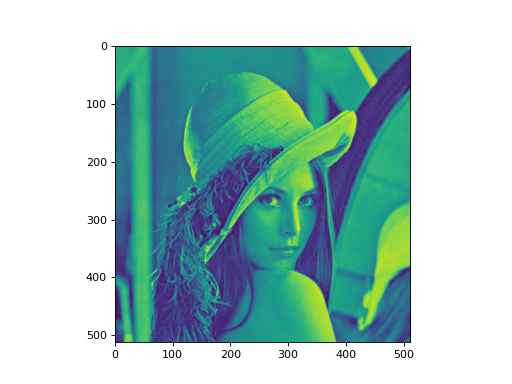# Color Space Conversions¶

New in version 0.9.6.

## Red-green-blue images¶

An RGB image is represented as a 3-dimensional array of shape `(h,w,3)`, where each pixel is represented by three values, red/green/blue.

For example, the classic lena image is a `(512,512,3)` array:

```import mahotas as mh
print(lena.shape)
```

We can convert it to greyscale as using `rgb2grey` (or `rgb2gray` if you prefer, both work). This conversion uses a visually realistic method (which weighs the green channel more heavily as human eyes are more sensitive to it). For example:

```import mahotas as mh
lenag = mh.colors.rgb2grey(lena)
```
```from pylab import imshow
import mahotas as mh
lenag = mh.colors.rgb2grey(lena)

imshow(lenag)
```We can also convert to sepia with `rgb2sepia`:

```lenas = mh.colors.rgb2sepia(lena)
```
```from pylab import imshow
import mahotas as mhMahotas can also convert to XYZ space and to the Lab space with `rgb2xyz` and `rgb2lab`, respectively.